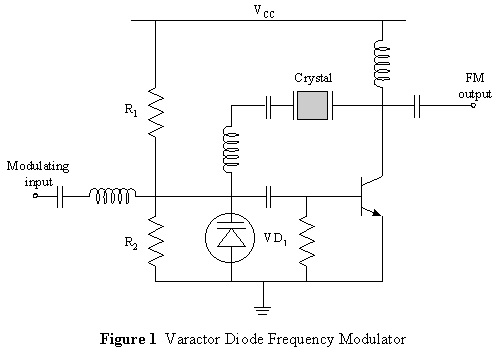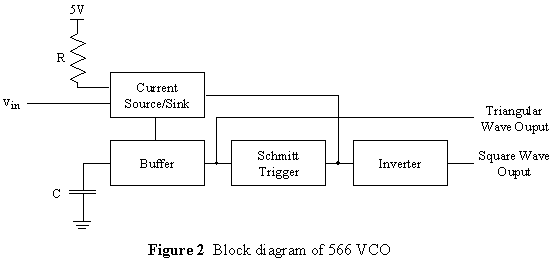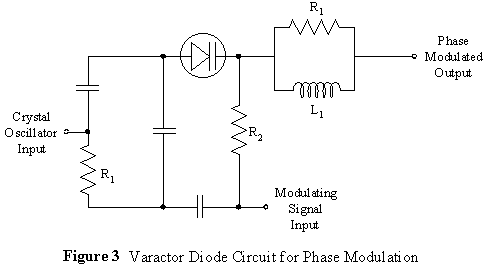# Varactor diodes

A collection of articles about varactor diodes

• Paraceiver; A 40m parametrically-pumped regenerative receiver

Varactors and Parametric Amplification

##### This negative resistance and amplification mentioned above has been obtained through energy supplied from an outside RF source.

FM modulators using varactors

There are two types of FM modulators - direct and indirect. Direct FM involves varying the frequency of the carrier directly by the modulating input. Indirect FM involves directly altering the phase of the carrier based on the input (this is actually a form of direct phase modulation.

Direct modulation is usually accomplished by varying a capacitance in an LC oscillator or by changing the charging current applied to a capacitor.

The first method can be accomplished by the use of a reverse biased diode, since the capacitance of such a diode varies with applied voltage. A varactor diode is specifically designed for this purpose. Figure 1 shows a direct frequency modulator which uses a varactor diode.This circuit deviates the frequency of the crystal oscillator using the diode. R1 and R2 develop a DC voltage across the diode which reverse biases it. The voltage across the diode determines the frequency of the oscillations. Positive inputs increase the reverse bias, decrease the diode capacitance and thus increase the oscillation frequency. Similarly, negative inputs decrease the oscillation frequency.

The use of a crystal oscillator means that the output waveform is very stable, but this is only the case if the frequency deviations are kept very small. Thus, the varactor diode modulator can only be used in limited applications.

The second method of direct FM involves the use of a voltage controlled oscillator, which is depicted in figure 2.The capacitor repeatedly charges and discharges under the control of the current source/sink. The amount of current supplied by this module is determined by vIN and by the resistor R. Since the amount of current determines the rate of capacitor charging, the resistor effectively controls the period of the output. The capacitance C also controls the rate of charging. The capacitor voltage is the input to the Schmitt trigger which changes the mode of the current source/sink when a certain threshold is reached. The capacitor voltage then heads in the opposite direction, generating a triangular wave. The output of the Schmitt trigger provides the square wave output. These signals can then be low-pass filtered to provide a sinusoidal FM signal.

The major limitation of the voltage controlled oscillator is that it can only work for a small range of frequencies. For instance, the 566 IC VCO only works a frequencies up to 1MHz.

A varactor diode circuit for indirect FM is shown in figure 3.The modulating signal varies the capacitance of the diode, which then changes the phase shift incurred by the carrier input and thus changes the phase of the output signal. Because the phase of the carrier is shifted, the resulting signal has a frequency which is more stable than in the direct FM case.